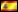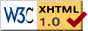Invited signatures

# Expression trees in C# 3.0

#####Versión en español

C# 3.0’s lambda expressions have two different facets of representation and usage, tightly related between them: as code and as data (in the form of expression trees, data structures capable of representing in an efficient manner the structure and, in consequence, the algorithm of evaluation of the expression).

### 1. Introduction

The end of the year has been busier than expected, and that has been the main reason behind the delay of this promised second installment. But, at last, here it is!

In our previous issue , we introduced C# 3.0’s lambda expressions and discussed how they have two different facets of representation and usage, tightly related between them: as code (in the form of anonymous methods, executable blocks of executable ILAsm code) and as data (in the form of expression trees, data structures capable of representing in an efficient manner the structure and, in consequence, the algorithm of evaluation of the expression). Then we placed more emphasis in the first facet, and now it is time to concentrate on expression trees.

### 2. Expression trees as a representation of expressions

As we have seen before, lambda expressions are compiled as code or data depending on the context where they are used. For instance, if we assign a lambda expression (for this example we have used an expression that implements the well-known formula for calculating the area of a circle) to a variable of a delegate type, like this:

`Func<double, double> circleArea = (radius) => Math.PI * radius * radius;`

the compiler will generate inline the corresponding ILAsm code, so that the previous definition is functionally equivalent to the following anonymous method assignment:

```Func<double, double> circleArea =

On the other hand, if we assign the lambda expression to a variable of the generic type Expression<T>, the compiler won’t translate it into executable code, but will instead generate an in-memory tree of objects that represents the structure of the expression itself. These data structures are known in C# 3.0 as expression trees. Continuing with the same example, if we use the lambda expression that calculates the area of a circle like this:

```static Expression<Func<double, double>> circleAreaExpr =

What we are expressing translates roughly into the following sequence of assignments:

```static ParameterExpression parm =

static Expression<Func<double, double>> circleAreaExpr =
Expression.Lambda<Func<double, double>>(
Expression.Multiply(
Expression.Constant(Math.PI),
Expression.Multiply(
parm,
parm)),
parm);```

The first of the previous assignments creates an object of the ParameterExpression class, which represents the only parameter (variable, in mathematical terms) of the lambda expression (function, again using the common jargon of mathematics).  The second sentence is where the expression tree is really built, using the object-parameter obtained in the previous step. Observe here the functional programming style used when defining an expression tree through code – a style that will become much more common with the release of C# 3.0 and LINQ (particularly LINQ To XML, the LINQ extension for the treatment of XML documents).

Once you have built an expression tree, it can be manipulated in the same way as any other .NET object – modified, serialized for persistent storage or transmission through the network, etc. Specifically, the Expression<T> class offers the means for compiling an expression tree “on the fly” into the ILAsm code necessary for its evaluation:

```Func<double, double> area = circleAreaExpr.Compile();

Console.WriteLine("The area of a circle with radius 5 is " + area(5));```

### 3. The expressions class hierarchy

The library classes that are needed in order to work with expression trees are implemented in the assembly System.Query.dll, and their namespace is System.Expressions (this can change as the product matures). The first thing to note is that there are two classes named Expression: the generic one we have used before, and another non-generic one, on which the previous one relies and that constitutes the real “power horse” that supplies all the machinery of representation of expressions. The generic class is of higher level, and imposes the strong type checking mechanism needed, for instance, in order to compile an expression tree into an anonymous method. The other one is a bit more type-relaxed, and relies more on features like reflection in order to allow a higher freedom of implementation. Normally, an object of the first class will be built from an instance of the second.

The non-generic version of Expression is similar to the typical classes obtained when implementing recursive data structures in object oriented programming languages. It’s an abstract class, and from it derives a whole bunch of other abstract as well as “concrete” classes used to represent the different types of elements that can appear in an expression. Some of the most common descendants of Expression are shown in the following table.

 Class Meaning LambdaExpression This class serves as “bridge” between the non-generic Expression class and the generic one, Expression. Often, the “manual” construction of an expression tree starts with the creation of an object of this type, as the samples in this article show. Its main properties are Body, which represents the body of the expression, and Parameters, the list of parameters it uses. ParameterExpression The parameters (variables) of an expression are represented through objects of this class. In order to evaluate an expression, values must be supplied for each of its parameters. The main property of this class is Name, which stands for the symbolic name of the parameter. ConstantExpression The constant values that appear in an expression are represented through objects of this class. Its main property is Value, which returns the (constant) value of the expression. UnaryExpression Though instances of this class we can represent expressions based in the application of a unary operator (like the change of sign or logical negation) to another, inner expression. The main property of this class is Operand, associated to the (only) operand in the expression. BinaryExpression Though instances of this class we can represent expressions based in the application of a binary operator (like sum, multiplication and many others) to another two expressions. The main properties of this class are Left y Right, which provide access to the left and right operand, respectively. MethodCallExpression Though instances of this class we can represent expressions built by means of a call to a method. The main properties of this class are Method (the metadata information associated to the method to be called); Object, the object to which the method call will be applied (null in case the method is static) and Parameters, a list of the expressions to be used as arguments to the method call. Note: There are more subclasses of Expression not described here.

The most important thing to note about these subclasses of Expression is that their constructors aren’t public; for this reason, in order to instantiate them it is necessary to use the static factory methods included in the Expression class, as shows the sentence in the sample code that builds the tree corresponding to the area of the circle. These factory methods, of course, include one or more parameters of type Expression, in order to allow the recursive nesting of expressions.

For another, more complex example, see how we could create in code the expression tree corresponding to the hypotenuse of the right triangle introduced in our previous installment:

```static ParameterExpression px = Expression.Parameter(typeof(double), "x");
static ParameterExpression py = Expression.Parameter(typeof(double), "y");
static ParameterExpression[] parms = { px, py };

static Expression<Func<double, double, double>> hypotenuseExpr2 =
Expression.Lambda<Func<double, double, double>>(
Expression.Call(
typeof(Math).GetMethod("Sqrt"),
null,
new Expression[] {
Expression.Multiply(px, px),
Expression.Multiply(py, py))
}),
parms);

static void Main(string[] args)
{

Console.WriteLine(hypotenuseExpr2);
// prints '(x, y) => Sqrt(Add(Multiply(x, x), Multiply(y, y)))'

Func<double, double, double> hypo = hypotenuseExpr2.Compile();
Console.WriteLine("Hypotenuse(3, 4) = " + hypo(3, 4));
}```

### 4. The perfect example

In my humble opinion, the perfect example in order to show the possibilities that C# 3.0 expression trees offer consists in the development of an interpreter of mathematical expressions. An expression interpreter is an application that accepts from the user a string that represents a mathematical expression (such as 2 * sin(x) * cos(x), for instance) and translates it into an internal representation that facilitates the further evaluation of the expression for different values of the variables (x in this case). An expression interpreter based in C# 3.0 expression trees would use expression trees for the internal representation of the mathematical expressions.

This article does not include an implementation of such an interpreter, just because of the fact that somebody has done it before me. While I was concentrated in other tasks (much less interesting, rest assured :-), MVP colleague Bart De Smet  has provided an excellent implementation in a series of blog entries, which can serve as a complementary reading to this article and I sincerely recommend.

### 5. Conclusions

Expression trees are another important new feature to be included in the next 3.0 version of C#. In this article we have shown how expression trees provide for an efficient data representation of lambda expressions (functions, ultimately), y how these trees can be transformed into code and evaluated when necessary. In a future installment we will describe how this possibility is exploited in LINQ (and specifically in LINQ To SQL) in order to postpone the evaluation of a LINQ query expression until the precise moment when all the information needed for its optimal execution is available.

The source code of the example can be downloaded from this site. In order to run it, the May 2006 LINQ Preview, available at , must be downloaded and installed.

### 6. References

1. C# 3.0 and LINQ related resources: http://msdn.microsoft.com/csharp/future/default.aspx.
2. Hernández, Octavio “What Orcas will bring: new features in C# 3.0”, published in dotNetManía Nº 24, March 2006 (in Spanish).
3. Hernández, Octavio “What Orcas will bring: the LINQ Project”, published in dotNetManía Nº 25, April 2006 (in Spanish).
4. Hernández, Octavio “Lambda expressions in C# 3.0”, in , October 2006.
5. De Smet, Bart “Dynamic Compilation Illustrated” (Parts I-IV), in  .

 Sample code (ZIP): File with sample code: octavio_Arboles.zip - 10.70 KB (MD5 checksum: E34D4CF72E330200C58D6C2940F4A40C)